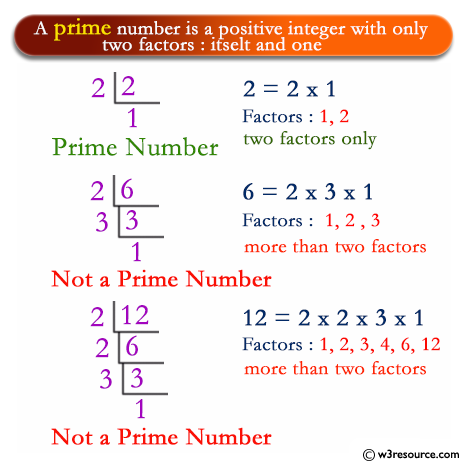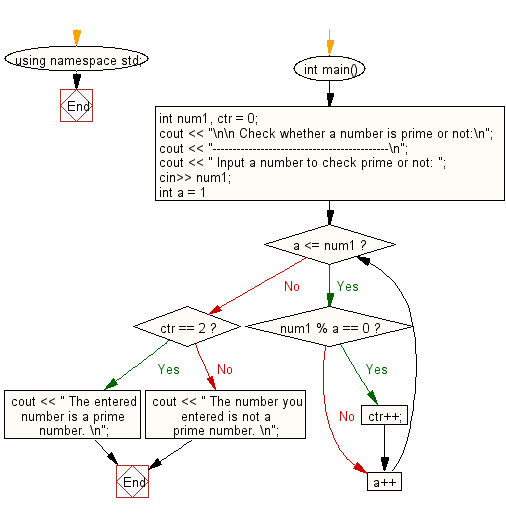﻿ C++ Exercises: Check whether a number is prime or not - w3resource

# C++ Exercises: Check whether a number is prime or not

## C++ For Loop: Exercise-5 with Solution

Write a program in C++ to check whether a number is prime or not.

Pictorial Presentation:Sample Solution :-

C++ Code :

``````#include <iostream>
using namespace std;
int main()
{
int num1, ctr = 0;
cout << "\n\n Check whether a number is prime or not:\n";
cout << "--------------------------------------------\n";
cout << " Input a number to check prime or not: ";
cin>> num1;
for (int a = 1; a <= num1; a++)
{
if (num1 % a == 0)
{
ctr++;
}
}
if (ctr == 2)
{
cout << " The entered number is a prime number. \n";
}
else {
cout << " The number you entered is not a prime number. \n";
}
}
``````

Sample Output:

``` Check whether a number is prime or not:
--------------------------------------------
Input a number to check prime or not: 13
The entered number is a prime number.
```

Flowchart:C++ Code Editor: